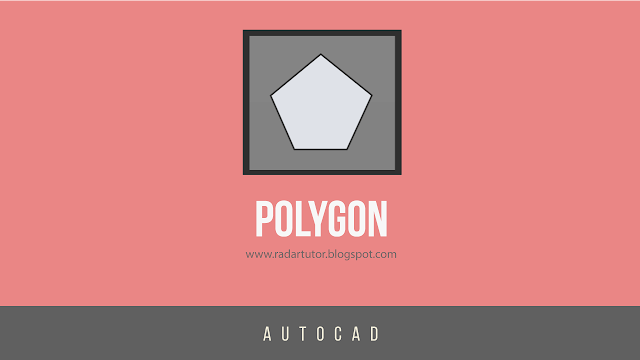## Social Items

Polygon functions to create multiple objects with equal lengths. The number of polygon sides that can be formed is at least 3 sides (triangles), while the most sides that can be formed are 1024 sides. A polygon can be formed with two methods, namely Inscribed in circle and Circumscribed about circle.

How to activate the polygon function in AutoCAD:
1. Commands Methode
Command: polygon
2. Toolbar Methode

The differences between Inscribed in circle and Circumscribed about circle

Creating Polygons with the Inscribed in circle Method :
1. Type "POLYGON" in the command bar, then enter.
2. Type the number of the polygon side, for example 5, then press enter.
3. Click the center point of the polygon.
4. Type I, then press enter.
5. Type the polygon radius, for example 4, then press enter.
Creating Polygons with the circumscribed in cycle method:
1. Type "POLYGON" in the command bar, then enter.
2. Type the number of the polygon side, for example 5, then press enter.
3. Click the center point of the polygon.
4. Type C, then press enter.
5. Type the polygon radius, for example 4, then press enter.

If you are interested in this information, you can join this website for FREE!Polygon functions to create multiple objects with equal lengths. The number of polygon sides that can be formed is at least 3 sides (triangles), while the most sides that can be formed are 1024 sides. A polygon can be formed with two methods, namely Inscribed in circle and Circumscribed about circle.

How to activate the polygon function in AutoCAD:
1. Commands Methode
Command: polygon
2. Toolbar Methode

The differences between Inscribed in circle and Circumscribed about circle

Creating Polygons with the Inscribed in circle Method :
1. Type "POLYGON" in the command bar, then enter.
2. Type the number of the polygon side, for example 5, then press enter.
3. Click the center point of the polygon.
4. Type I, then press enter.
5. Type the polygon radius, for example 4, then press enter.
Creating Polygons with the circumscribed in cycle method:
1. Type "POLYGON" in the command bar, then enter.
2. Type the number of the polygon side, for example 5, then press enter.
3. Click the center point of the polygon.
4. Type C, then press enter.
5. Type the polygon radius, for example 4, then press enter.

If you are interested in this information, you can join this website for FREE!